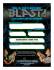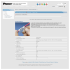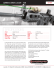# Laser Physics Homework 7, Due Date

## Transcription

Laser Physics Homework 7, Due Date
```Laser Physics: PHYS 5734/4734
Spring 2015, Homework Set - 7
Due Date: Friday, April 30, 5 pm
Some cool concepts (Problems 1 and 2):
1. Given that the steady-state (average) photon number in the laser cavity, when spontaneous emission is included, is given by


s
2
qs 
4r
1
1
qss =
+
+ ,
r−1−
r−1−
2
qs
qs
qs
which can be approximated to be
r
qs
4r
2
(r − 1) + (r − 1) +
,
qss ≈
2
qs
where r = N (o) /Nth is the pump ratio, qs = γ2 /K is the saturation photon number. In
writing this solution we have dropped the unphysical solution (which gives a negative
value for q).
(a) Plot (not sketch) both expressions for qss as a function of pump ratio r over the
range r = 0.90 (10% below threshold) to r = 1.10 (10% above threshold) for qs = 108 .
Hint: You may have to use a log scale for the photon number.
(b) In your calculations below, take γ2 ≈ γ21 ≈21rad , which is a good approximation
for a large class of lasers. Also recall the definitions of saturation photon number qs ,
spontaneous emission per mode K = γ21rad /p where p is the number of modes that
couple to the atomic transition, pump ratio r = N (0) /Nth , and Nth = γc /K. Show
that
(i) below threshold (r 1) only a fraction p1 of spontaneously emitted photons is
channeled into the cavity mode
(ii) above threshold (r 1), almost all spontaneously emitted photons are channeled
into the cavity mode.
Hint: Compare the steady-state photon number in the cavity to the number of spontaneously emitted photons in a cavity life time τc = 1/γc .
(c) Consider a high-Q, two mirror cavity with one mirror nearly perfectly reflecting and
the other with 2% transmission and negligible other losses. Take the separation between the mirrors to be 50 cm. If, on average, the laser cavity has one photon in the
steady-state (qss = 1) (presumably because it is being operated below threshold) how
many photons does the laser emit per second?
2. Relaxation oscillations: Investigate the possibility of relaxation oscillations in the
following systems and find their life-time, frequency and period.
1
(a) A typical GaAs injection laser has a cavity length of 300 µm. Its end faces are cleaved
and act as mirrors with Fresnel reflectivity R = 30% (n = 3.35). Distributed loss
coefficient is 60 cm−1 . Assuming laser operation 50% above threshold and upper
state lifetime of 3 ns, find the frequency and lifetime of relaxation oscillations.
(b) A He:Ne laser oscillating 50% above threshold has an upper level lifetime of 50 ns.
Assume a cavity of length 50.0 cm, output coupling of 1% and negligible other losses.
Discuss the possibility of relaxation oscillations.
3. Q-switching in Nd:YAG (r = 2.0): A Nd:YAG laser operating at wavelength
λ = 1.064 µm consists of an 18-cm long standing wave cavity containing a 5.0 cm
long Nd:YAG rod ( refractive index n = 1.82, upper level lifetime 230 µs) with an
effective cross-sectional area of 0.20 cm2 . The laser is operated in the Q-switched
regime. Threshold inversion density for the laser is ∆Nth = 4.35 × 1017 cm−3 and it is
pumped 2.0 times above threshold. Given that R1 ≈ 1, R2 = 30%, round-trip internal
loss Li = 33% and the effective cavity length Le = 18 + (1.82 − 1)5.0 = 22.1 cm.
(a) What fraction of energy stored initially in the inversion, is converted into light?
What is the total pulse energy output from the laser?
(b) Calculate the peak photon number in the cavity and the peak output power from
the laser? What is the pulse width?
4. Mode-locking
(a) Ar-ion gas laser:
A 100 cm long Ar-ion laser, with a Doppler linewidth
(FWHM) of 3.5 GHz, has Brewster windows on the gain tube to ensure oscillation in
single polarization. It is pumped so that the unsaturated line center gain is 4 times the
threshold value. How many TEM00 modes could oscillate simultaneously? If all the
modes were locked together, what would the repetition rate and width of the pulses
be? This pulse width is rather large. Dye laser and solid state lasers can usually
produce much shorter mode-locked pulses [see part (b)].
(b) Dye laser: Rhodamine 6G dye laser that you used in the lab has a homogeneously broadened gain line, tunable, approximately, over the wavelength range 570
nm - 600 nm. Assuming a cavity length of L = 1.5 m, and assuming the oscillation bandwidth to be equal the gain linewidth, calculate the expected pulse width
when the laser is mode-locked by using an acousto-optic modulator. What frequency
would be needed to drive the AOM for mode-locking? What would be the expected
pulse width for a laser with an inhomogeneously broadened gain profile of the same
oscillation bandwidth?
2
```

### Nanocomp Pattern generators, PDF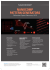### Homework #11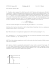### Laser Engraving - Source International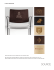### INVITATION TO: - Diowave Laser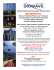### GASGLASS LASER NEW GAS FILL ANALYZER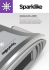### laser pointer pen sale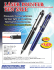### SPEcificAtionS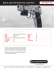### Here - Ultrazone